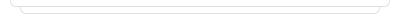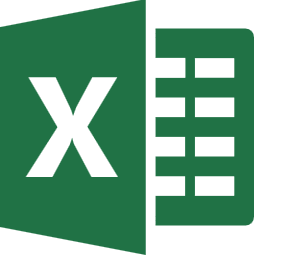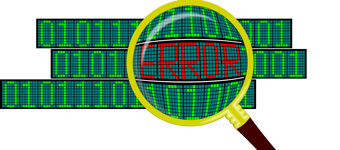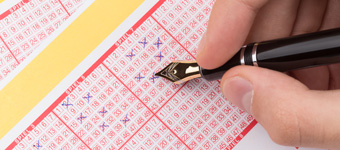Free Excel Training and Courses### Practice: VLOOKUP-IV

Making more practice by using lookup functions.### Practice: VLOOKUP-IV

Making more practice by using lookup functions.### Practice: VLOOKUP-II

Making more practice by using lookup functions.### Practice: VLOOKUP-II

Making more practice by using lookup functions.### iPhone vs Samsung

Checking formulas results with Iphone-Samsung sales.### iPhone vs Samsung

Checking formulas results with Iphone-Samsung sales.### Error Handling

Correcting value errors.### Error Handling

Correcting value errors.### Electronic Sales

Using formulas for "Electronic Sales" operations.### Electronic Sales

Using formulas for "Electronic Sales" operations.### Practice: VLOOKUP-I

Making more practice by using lookup functions.### Practice: VLOOKUP-I

Making more practice by using lookup functions.### Staff Data

Getting some information with VLOOKUP on "Employee Data"### Staff Data

Getting some information with VLOOKUP on "Employee Data"### Practice: VLOOKUP-III

Making more practice by using lookup functions.### Practice: VLOOKUP-III

Making more practice by using lookup functions.### Practice: VLOOKUP-V

Making more practice by using lookup functions.### Practice: VLOOKUP-V

Making more practice by using lookup functions.### Win a lottary ticket### Win a lottary ticket### Practice: VLOOKUP-IV

Making more practice by using lookup functions.### Practice: VLOOKUP-II

Making more practice by using lookup functions.### iPhone vs Samsung

Checking formulas results with Iphone-Samsung sales.### Error Handling

Correcting value errors.### Electronic Sales

Using formulas for "Electronic Sales" operations.### Practice: VLOOKUP-I

Making more practice by using lookup functions.### Staff Data

Getting some information with VLOOKUP on "Employee Data"### Practice: VLOOKUP-III

Making more practice by using lookup functions.### Practice: VLOOKUP-V

Making more practice by using lookup functions.### Win a lottary ticket